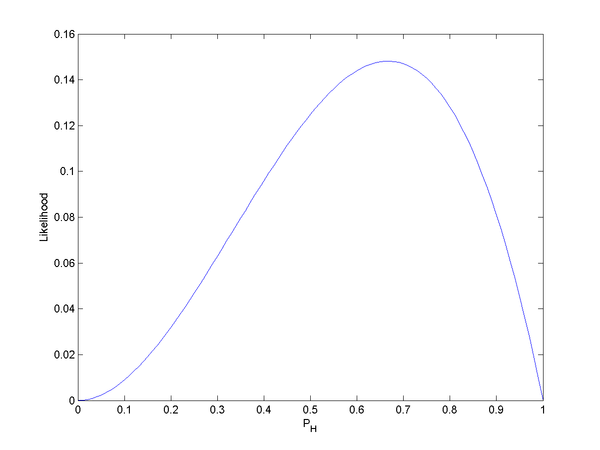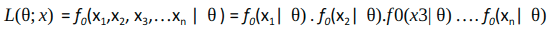# 似然函数 | 最大似然估计 | likelihood | maximum likelihood estimation | R代码

## 似然函数 | 似然值

wiki：在数理统计学中，似然函数是一种关于统计模型中的参数的函数，表示模型参数中的似然性。

（传统的贝叶斯定理的适用范围很广，是高度的总结推广，在似然函数里就不要过于推广了）

L(θ|x) 和 f(θ|x)有什么本质的区别？ 一个问题看你懂不懂条件概率和似然值wiki案例：图2. 三次投掷中头两次正面朝上，第三次反面朝上时的似然函数。可以看到似然函数的积分并不等于1，需要关注的只是大小的相对变化.

## 最大似然估计

An Introductory Guide to Maximum Likelihood Estimation (with a case study in R)

Given the observed data and a model of interest, we need to find the one Probability Density Function/Probability Mass Function (f(x|θ)), among all the probability densities that are most likely to have produced the data.

To solve this inverse problem, we define the likelihood function by reversing the roles of the data vector x and the (distribution) parameter vector θ in f(x| θ), i.e.,
L(θ;x) = f(x| θ)• Newton’s method,
• Fisher scoring,
• various conjugate gradient-based approaches,
• steepest descent,
• Nelder-Mead type (simplex) approaches,
• BFGS

### MLE using R

# Modelling Poisson Distrinution using Singla variables
library(dplyr)
library(ggplot2)
library(caret)
library(ModelMetrics)
library(stats4)
#Read training data

# X <- read.csv("Train_Tickets.csv", header = T)
X <- read.table("https://s3-ap-south-1.amazonaws.com/av-blog-media/wp-content/uploads/2018/07/Train_Tickets.csv", header = T, sep = ",")

#Call Formatfile fun to calculate derived variables
Y <- Formatfile(X)

#Plot density of the sale
hist(Y$Count, breaks = 50,probability = T ,main = "Histogram of Count Variable") lines(density(Y$Count), col="red", lwd=2)

X$dt <- as.POSIXct(X$Datetime, format="%d-%m-%Y %H:%M")

#Plot of Sale over time
ggplot(data=X,aes(x=dt, y=Count)) +
geom_path(colour="blue") +
ylab("Count of Tickets Sold") +
xlab("Date") +
labs(title="Ticket Sale over time")

#Partition data into test and train set
set.seed(200)
idx <- createDataPartition(Y$Count, p=0.25,list=FALSE) #Negative log likelihood Function using Poisson Distribution nll <- function(theta0,theta1) { x <- Y$age[-idx]
y <- Y$Count[-idx] mu = exp(theta0 + x*theta1) -sum(y*(log(mu)) - mu) } #Parameter Estimation est <- stats4::mle(minuslog=nll, start=list(theta0=2,theta1=0)) summary(est) #Prediction on test set and RMSE pred.ts <- (exp(coef(est)['theta0'] + Y$age[idx]*coef(est)['theta1'] ))
rmse(pred.ts, Y$Count[idx]) #86.95227 #Comparison with standard linear model lm.fit <- lm(log(Count)~age, data=Y[-idx,]) pred.lm <- predict(lm.fit, Y[idx,]) rmse(exp(pred.lm), Y$Count[idx])
#93.77393

Formatfile <- function(File){
## Separate columns and format them
File1 <- File
File1$Datetime <- as.character(File1$Datetime)
File1 <- mutate(File1, tdate = substr(Datetime, 1,10), thr = substr(Datetime, 12,13))
## convert Hour as factor
File1$thr <- as.factor(File1$thr)
## FInd Weekday of the date
Dt <- as.Date("25-08-2012", "%d-%m-%Y")
File1 <- mutate(File1, weekday = weekdays(as.Date(File1$tdate, "%d-%m-%Y")), age = -difftime(Dt, as.Date(File1$tdate, "%d-%m-%Y"), units = "weeks"),
Mon = months(as.Date(File1$tdate, "%d-%m-%Y")), Day = substr(File1$tdate,1,2))

File1$weekday <- as.factor(File1$weekday)
File1$Mon <- factor(File1$Mon)
File1$Day <- factor(as.character(File1$Day))
File1$age <- as.numeric(File1$age)
## Remove Date coulmns
File1 <- select(File1, thr, weekday, age, Mon, Day, Count)

## Return File1
File1
}


f(x|θ)，按照定义就是当我们已知θ=p=0.5时，x=5出现的概率，根据二项分布；计算可得：f(x=2|θ=0.5) = 0.25

f(θ|x)，按照定义就是当我们已知x=2时，p=0.5出现的概率，此时的限定空间是x=2；f(θ=0.5|x=2) ，这就是贝叶斯的后验概率。

f(θ|x) = f(x|θ) * f(θ) / f(x)，一定要好好理解这个公式。

L(θ|x)，按照定义就是给定数据x=5时，p=0.4~0.5出现的概率（显然不能这么定义，这就和f(θ|x)一模一样了）。

• 同一次随机试验下的随机事件，A1,B1
• 不同随机试验下的随机事件，A1,A2

P(A, B)和P(A|B)本质的区别？P(AB) ，没错最终的效果是一样的A和B都会发生，但是出发点不一样，一个是全集，一个是B空间。

posted @ 2019-05-15 21:11 Life·Intelligence 阅读(...) 评论(...) 编辑 收藏
TOP